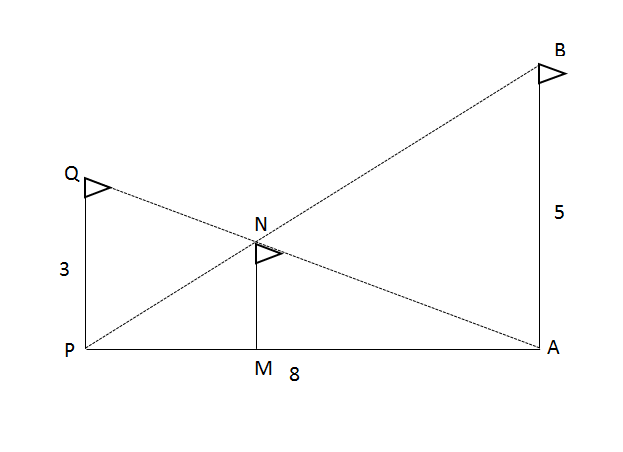# CAT Quantitative Aptitude Questions | Geometry Questions for CAT - Triangles

###### CAT Questions | CAT Geometry Questions | Triangles

The question is from the topic triangles. This question is about similar triangles which are formed by the flag of the medal winning countries. CAT Geometry questions are heavily tested in CAT exam. Make sure you master Geometry problems. It tests our understanding of the concept similar triangles.

Question 44: The Olympics committee came up with a new rule. The flag of the gold medal winning team would be hoisted to the right (AB) at 5m. The flag of silver medal winning team would be hoisted to the left (PQ) at a height of 3m. The flag (MN) of bronze medal winning team would be hoisted at the point of intersection of the line joining the top of each of AB and PQ to the foot of other, as shown in the figure below. A and P are 8m apart. In a wrestling event, India won the bronze medal. Find the height at which the Indian flag was hoisted.1. 2 m
2. $$frac{5}{2}\\$ m 3. $\frac{5}{8}\\$ m 4. $\frac{15}{8}\\$ m ## 🎉Get Upto ₹10,000 off on our CAT 23/24 courses.🎉Flat ₹6000 off on IPM courses.Valid till 30th September 2023!★ Register now ## Best CAT Coaching in Chennai #### CAT Coaching in Chennai - CAT 2022Limited Seats Available - Register Now! ### Explanatory Answer ##### Method of solving this CAT Question from Triangles: Understanding of similar triangles would help here. From the figure, ΔPAB ~ ΔPMN and ΔAMN~ΔAPQ ΔPAB ~ ΔPMN Therefore, $\frac{PM}{PA}\\$ = $\frac{MN}{AB}\\$ => $\frac{PM}{8}\\$ = $\frac{MN}{5}\\$…………………$1)
Also, ΔAMN~ΔAPQ
Therefore, $$frac{AM}{AP}\\$ = $\frac{MN}{PQ}\\$ => $\frac{AM}{8}\\$ = $\frac{MN}{3}\\$…………………$2)